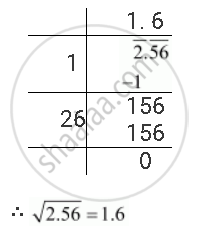Share

# Find the Square Root of the Following Decimal Number 2.56 - CBSE Class 8 - Mathematics

ConceptSquare Roots of Decimals

#### Question

Find the square root of the following decimal number

2.56

#### Solution

The square root of 2.56 can be calculated as follows.Is there an error in this question or solution?

#### APPEARS IN

NCERT Solution for Mathematics Textbook for Class 8 (2018 to Current)
Chapter 6: Squares and Square Roots
Ex. 6.40 | Q: 3.1 | Page no. 108
Solution Find the Square Root of the Following Decimal Number 2.56 Concept: Square Roots of Decimals.
S